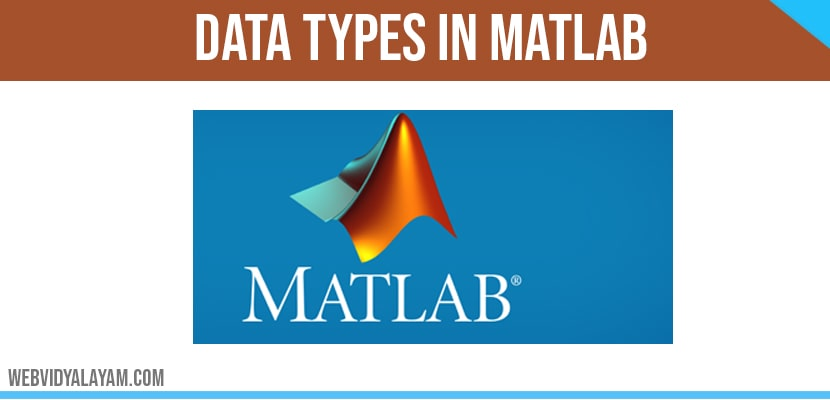# Data Types in Matlab

Class require different storage amounts logical value or 8-bit integer is the smallest of all and it require 1byte.

## INTEGER:

• Use for signed and unsigned whole numbers.
• Most efficiently use of memory.
• intmin and intmax useful to show the range of values.
• Chooses from 4 different sizes (8, 16, 32, and 64 bits).

Example: int8, uint8, int16, uint16, int32, uint32, int64, uint64

In matlab it as four signed and unsigned integer classes. Signed work for positive and negative values but wide range of numbers cannot represented as unsigned type.

## LOGICAL OPERATIONS:

• Itconsist of two values i.e., true or false.
• It is used to test state or for relational condition.
• It useful for array indexing.
• Inefficiency of two dimensional arrays.

## CHARACTER AND STRING:

• Data type into text.
• Native or Unicode.
• Which is used to convert to/from numeric.
• For multiple strings.

## FLOATING POINT NUMBER:

• It is default numerical type in matlab.
• To show range of values it uses realmin and realmax.
• Two-dimensional array can sparse.
• Floating point number is represented in Double and single precision in matlab.

In matlab default is double but using some techniques we convert into single precision with simple conversion.

## STRUCTURES:

• It require more memory for overhead.
• Method of passing function arguments.
• It can access one or all fields in single operation.

## CELL ARRAYS:

• Cells useful for storing varying classes and size of array.
• It requires more memory for overhead.
• In order to handle elements similarly as numeric or logical arrays.
• To package data as you want it allows you the freedom.

## TABLES:

• Rectangular container will manipulate the mixed-type and column-oriented data.
• Row names and variable names will identify contents.
• We can use Table Properties in order to store metadata such as variable units.
• In order to handle elements similarly as numeric or logical arrays.
• We can access data in numeric or named index form.

## FUNCTION HANDLES:

• Pointer to a function.
• Enables passing a function to another function.
• It used to call functions outside for usual scope.